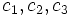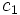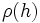# Size-degree-weighted characters are algebraic integers

## Statement

### For an algebraically closed field of characteristic zero

Suppose$k$ is an algebraically closed field of characteristic zero, and$G$ is a finite group. Let$\rho$ be an irreducible linear representation of$G$ over$k$, and$\chi$ be the character corresponding to$\rho$. Let$c$ be a conjugacy class in$G$ and$g \in c$ be an element. Denote the identity element of$G$ by$1$. Then:$\frac{|c|\chi(g)}{\chi(1)}$

is an algebraic integer.

Note that both aspects: irreducibility of the representation and the fact that we are working over an algebraically closed field of characteristic zero, are required. Getting rid of either condition makes the statement false.

Note: When the symbol$1$ appears as an input to a representation or a character, it refers to the identity element of$G$. When it appears as the output of a character, or in another context, it refers to the real number$1$.

### For a splitting field

We can weaken the condition on$k$ somewhat: instead of requiring it to be an algebraically closed field of characteristic zero, it suffices to require$k$ to be a Splitting field (?) for$G$. In particular, any sufficiently large field for$G$ will do.

## Related facts

### Applications and further results

Application name Full statement Intermediaries (if indirect application) Other facts used for the application How does it use this fact?
Degree of irreducible representation divides group order For a finite group and an irreducible linear representation over any splitting field of characteristic zero, the degree of the irreducible representation divides the order of the group. -- character orthogonality theorem, characters are algebraic integers The quotient of the size of the group by the degree of the irreducible representation is shown to be an algebraic integer, by writing it as a summation (using the character orthogonality theorem) and showing that the summands are all algebraic integers.
Zero-or-scalar lemma For a finite group and an irreducible linear representation over any splitting field of characteristic zero, if a conjugacy class size is relatively prime to the degree, either the character value at the conjugacy class is zero or the elements of the conjugacy class go to scalars. -- characters are algebraic integers, element of finite order is semisimple and eigenvalues are roots of unity We show that the quotient of the character value at the conjugacy class to the degree of the representation is an algebraic integer, and then use some bounding techniques to show that it is either zero or must be of norm 1.
Conjugacy class of prime power size implies not simple If a finite group has a conjugacy class whose size is a power of a prime (and not just 1), then the group is not a simple group via zero-or-scalar lemma characters are algebraic integers, column orthogonality theorem We use the column orthogonality theorem and derive a contradiction from simplicity by showing that a non-integer rational must be an algebraic integer.
Order has only two prime factors implies solvable (also known as Burnside's$p^aq^b$-theorem) If the order of a finite group has at most two distinct prime factors, then it is solvable. via conjugacy class of prime power order implies not simple (too indirect for mention) (too indirect for mention)

### Breakdown for a field that is not algebraically closed and is not a splitting field

Further information: cyclic group:Z3

Let$G$ be the cyclic group of order three and$\R$ be the field.$G$ has an irreducible two-dimensional linear representation over$\R$ given by rotation by multiples of$2\pi/3$. For a non-identity element$g$ of$G$,$\chi(g) = -1$ for the corresponding character, while$\chi(1) = 2$. Thus, the expression works out to$-1/2$, which is not an algebraic integer.

### Breakdown for a representation that is not irreducible

The same example as the above (the one for breakdown over a field that is not algebraically closed) works. Specifically, the irreducible representation over$\R$ can be viewed as a reducible representation over$\mathbb{C}$.

## Proof

The proof is based on the idea of the convolution algebra on conjugacy classes.

### Description of the convolution algebra on conjugacy classes

Let$C(G,\mathbb{Z})$ be a$\mathbb{Z}$-subalgebra of the group ring$\mathbb{Z}(G)$ defined as follows: as a group, it is the free Abelian group on all indicator class functions for conjugacy classes. In other words, for each conjugacy class, we have a free generator that corresponds to the sum of elements of that conjugacy class.

The structure constant for multiplication of elements of$C(G,\mathbb{Z})$ is defined as follows: given conjugacy classes$c_1, c_2, c_3$, the coefficient of the$c_3$-indicator function in the product of the$c_1$-indicator function and the$c_2$-indicator function is the number of ways of writing$g_1g_2 = a$ where$g_i \in c_i$, and$a$ a fixed element of$c_3$.

Note that all the structure constants are integers.

### A homomorphism from this convolution algebra to the matrix ring

The representation$\rho$ gives rise to a homomorphism from$C(G,\mathbb{Z})$ to the matrix ring$M_n(k)$. The indicator function for a conjugacy class$c$ goes to the matrix given by:$\sum_{g \in c} \rho(g)$.

This sum commutes with$\rho(h)$ for all$h$, and thus, by Schur's lemma, the sum is a scalar matrix. The trace of the sum is$|c|\chi(g)$, so the sum must be a scalar matrix with scalar entry:$\frac{|c|\chi(g)}{\chi(1)}$.

Thus, the set of scalar matrices with entries described as above additively generate a group that is a ring under multiplication. The structure constants for this ring are the same as the structure constants for the convolution algebra. A result from algebraic number theory now tells us that this forces the entire ring to be a ring of algebraic integers, and in particular, the generating elements are algebraic integers.